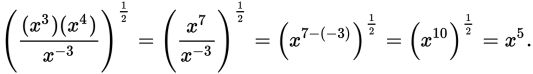# SAT Math Multiple Choice Question 330: Answer and Explanation

### Test Information

Question: 330

15. Which of the following is equivalent to the expression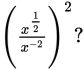• A. x2
• B.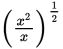• C.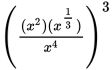• D.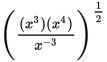Explanation:

D

Difficulty: Hard

Category: Passport to Advanced Math / Exponents

Strategic Advice: Being able to fluently use the rules of exponents will come in handy on Test Day. For this question, use these rules: when you raise a power to a power, you multiply the exponents, and when you divide with exponents, you subtract them.

Getting to the Answer: Distribute the 2 outside the parentheses to the exponent in the numerator and in the denominator: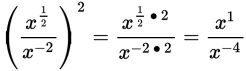Now, subtract the exponents: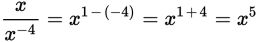Unfortunately, x5 is not one of the answer choices, so look for an answer choice that is also equivalent to x5. You can eliminate A right away, and the exponents in B look too small, so start with C, which simplifies to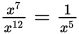and is therefore not correct. Choice (D) is correct because: